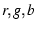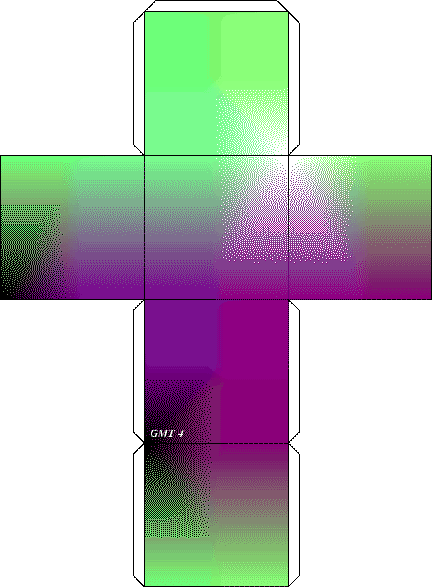Next: 7.12 Optimal triangulation of Up: 7. Cook-book Previous: 7.10 A geographical bar   Contents   Index

# 7.11 Making a 3-D RGB color cube

In this example we generate a series of 6 color images, arranged in the shape of a cross, that can be cut out and assembled into a 3-D color cube. The six faces of the cube represent the outside of the R-G-B color space. On each face one of the color components is fixed at either 0 or 255 and the other two components vary smoothly across the face from 0 to 255. The cube is configured as a right-handed coordinate system with x-y-z mapping R-G-B. Hence, the 8 corners of the cube represent the primaries red, green, and blue, plus the secondaries cyan, magenta and yellow, plus black and white.

The method for generating the 6 color faces utilizes $AWK in two steps. First, a z-grid is composed which is 256 by 256 with z-values increasing in a planar fashion from 0 to 65535. This z-grid is common to all six faces. The color variations are generated by creating a different color palette for each face using the supplied$AWK script rgb_cube.awk. This script generates a cpt'' file appropriate for each face using arguments for each of the three color components. The arguments specify if that component () is to be held fixed at 0 or 255, is to vary in x, or is to vary in y. If the color is to increase in x or y, a lower case x or y is specified; if the color is to decrease in x or y, an upper case X or Y is used. Here is the shell script and accompanying $AWK script to generate the RGB cube: #!/bin/csh # GMT EXAMPLE 11 # #$Id: job11.csh,v 1.7 2004/04/10 17:19:14 pwessel Exp $# # Purpose: Create a 3-D RGB Cube # GMT progs: gmtset, grdimage, grdmath, pstext, psxy # Unix progs:$AWK, rm
#
# First create a Plane from (0,0,0) to (255,255,255).
# Only needs to be done once, and is used on each of the 6 faces of the cube.
#

grdmath -I1 -R0/255/0/255 Y 256 MUL X ADD = rgb_cube.grd

#
# For each of the 6 faces, create a color palette with one color (r,g,b) fixed
# at either the min. of 0 or max. of 255, and the other two components
# varying smoothly across the face from 0 to 255.
#
# This uses $AWK script "rgb_cube.awk", with arguments specifying which color # (r,g,b) is held constant at 0 or 255, which color varies in the x-direction # of the face, and which color varies in the y-direction. If the color is to # increase in x (y), a lower case x (y) is indicated; if the color is to # decrease in the x (y) direction, an upper case X (Y) is used. # # Use grdimage to paint the faces and psxy to add "cut-along-the-dotted" lines. # gmtset TICK_LENGTH 0 COLOR_MODEL rgb pstext -R0/8/0/11 -Jx1i < /dev/null -P -U"Example 11 in Cookbook" -K >! example_11.ps$AWK -f rgb_cube.awk r=x g=y b=255 < /dev/null >! rgb_cube.cpt
grdimage rgb_cube.grd -Crgb_cube.cpt -JX2.5i/2.5i -R0/255/0/255 -K -O -X2i -Y4.5i -B256wesn \
>> example_11.ps

$AWK -f rgb_cube.awk r=255 g=y b=X < /dev/null >! rgb_cube.cpt grdimage rgb_cube.grd -Crgb_cube.cpt -J -K -O -X2.5i -B256wesn >> example_11.ps$AWK -f rgb_cube.awk r=x g=255 b=Y < /dev/null >! rgb_cube.cpt
grdimage rgb_cube.grd -Crgb_cube.cpt -J -K -O -X-2.5i -Y2.5i -B256wesn >> example_11.ps

psxy -W0.25pto -J -R -K -O -X2.5i << END >> example_11.ps
0 0
20 20
20 235
0 255
END

psxy -W0.25pto -J -R -K -O -X-2.5i -Y2.5i << END >> example_11.ps
0 0
20 20
235 20
255 0
END

psxy -W0.25pto -J -R -K -O -X-2.5i -Y-2.5i << END >> example_11.ps
255 0
235 20
235 235
255 255
END

$AWK -f rgb_cube.awk r=0 g=y b=x < /dev/null >! rgb_cube.cpt grdimage rgb_cube.grd -Crgb_cube.cpt -J -K -O -Y-2.5i -B256wesn >> example_11.ps$AWK -f rgb_cube.awk r=x g=0 b=y < /dev/null >! rgb_cube.cpt
grdimage rgb_cube.grd -Crgb_cube.cpt -J -K -O -X2.5i -Y-2.5i -B256wesn >> example_11.ps

echo "10 10 14 0 Times-BoldItalic BL GMT 4" | pstext -J -R -Gwhite -K -O >> example_11.ps

psxy -W0.25pto -J -R -K -O -X2.5i << END >> example_11.ps
0 0
20 20
20 235
0 255
END

psxy -W0.25pto -J -R -K -O -X-5i << END >> example_11.ps
255 0
235 20
235 235
255 255
END

$AWK -f rgb_cube.awk r=x g=Y b=0 < /dev/null >! rgb_cube.cpt grdimage rgb_cube.grd -Crgb_cube.cpt -J -K -O -X2.5i -Y-2.5i -B256wesn >> example_11.ps psxy -W0.25pto -J -R -K -O -X2.5i << END >> example_11.ps 0 0 20 20 20 235 0 255 END psxy -W0.25pto -J -R -O -X-5i << END >> example_11.ps 255 0 235 20 235 235 255 255 END \rm -f rgb_cube.cpt rgb_cube.grd .gmtcommands4 .gmtdefaults4  The$AWK script rgb_cube.awk is as follows:

#    $Id: rgb_cube.awk,v 1.1.1.1 2000/12/28 01:23:45 gmt Exp$
END{
z=-.5;

if(r=="X" || g=="X" || b=="X"){
xl=255; xr=0; xd=-255;
}else{
xl=0; xr=255; xd=255;
}

if(r=="Y" || g=="Y" || b=="Y"){
yb=255; yt=-1; yd=-1;
}else{
yb=0; yt=256; yd=1;
}

for(y=yb; y!=yt; y+=yd){

x=xl;

if(r=="x" || r=="X"){
if(g=="y" || g=="Y"){
printf("%7.1f %3d %3d %3d " ,z,x,y,b);
x+=xd; z+=256;
printf("%7.1f %3d %3d %3d\n",z,x,y,b);
}else{
printf("%7.1f %3d %3d %3d " ,z,x,g,y);
x+=xd; z+=256;
printf("%7.1f %3d %3d %3d\n",z,x,g,y);
}

}else if(g=="x" || g=="X"){
if(r=="y" || r=="Y"){
printf("%7.1f %3d %3d %3d " ,z,y,x,b);
x+=xd; z+=256;
printf("%7.1f %3d %3d %3d\n",z,y,x,b);
}else{
printf("%7.1f %3d %3d %3d " ,z,r,x,y);
x+=xd; z+=256;
printf("%7.1f %3d %3d %3d\n",z,r,x,y);
}

}else{
if(r=="y" || r=="Y"){
printf("%7.1f %3d %3d %3d " ,z,y,g,x);
x+=xd; z+=256;
printf("%7.1f %3d %3d %3d\n",z,y,g,x);
}else{
printf("%7.1f %3d %3d %3d " ,z,r,y,x);
x+=xd; z+=256;
printf("%7.1f %3d %3d %3d\n",z,r,y,x);
}
}
}
exit;
}


The cube can be viewed in Figure 7.11.Next: 7.12 Optimal triangulation of Up: 7. Cook-book Previous: 7.10 A geographical bar   Contents   Index
Paul Wessel 2006-01-01